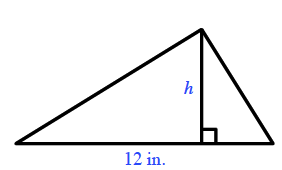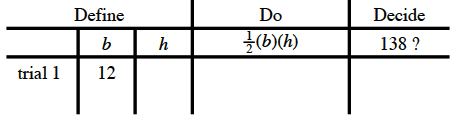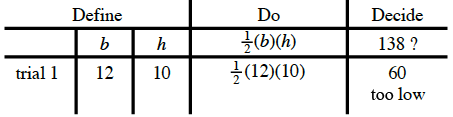### Home > MC2 > Chapter 5 > Lesson 5.3.2 > Problem5-102

5-102.

Draw a triangle. Choose one side as the base, and label it $12$ inches. Draw the height perpendicular to the base. If the area of the triangle is $138$ square inches, what is the height? You may find it helpful to use the 5-D Process.Possible 5-D table.

Pick a height number for trial If $h = 10$:For trial 2, use a larger height number.